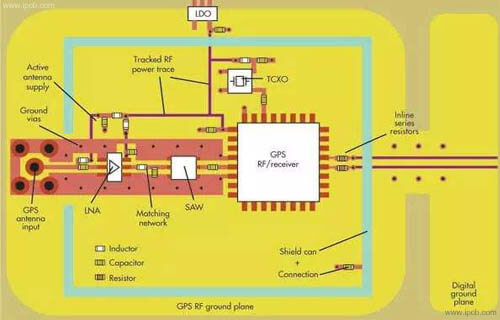전문 고주파 회로 기 판,고속 회로 기 판,IC 패 키 징 기 판,반도체 테스트 판,HDI 회로 기 판,소프트 하 드 결합 판,양면 다 층 판,PCB 디자인 및 PCBA 제조

### 빠 른 가격 획득.

sales@ipcb.com# PCB기술## PCB기술

Principle of high frequency circuit and function of high frequency PCB

Circuit principle

Circuit principle is divided into electronic circuit and "power circuit", which is generally referred to as "strong current" or "weak current" circuit; it refers to the "direction" path of the circuit in the aspects of manufacture, work and maintenance, as well as the size, direction of current path, control mode of current and other relevant conditions and information. If it is represented by drawings and diagrams, it is called circuit origin Diagram. So the course of circuit principle should be about these knowledge. The principle of high frequency circuit is based on the basic knowledge of electricity to explain the circuit knowledge of the current with "extremely short cycle" and high frequency in the alternating current (AC) category.High frequency circuit

Composition and principle of High frequency circuit

The passive components or networks in high-frequency circuit mainly include high-frequency oscillation (resonance) circuit, high-frequency transformer, resonator and filter, etc. they complete the functions of signal transmission, frequency selection and impedance transformation.

High frequency oscillation circuit is a passive network widely used in high-frequency circuit. It is also the main component of high-frequency amplifier, oscillator and various filters. It can complete impedance transformation, signal selection and other tasks in the circuit, and can be directly used as a load.

The oscillation circuit is composed of inductance and capacitance. The oscillation circuit with only one circuit is called simple oscillation circuit or single oscillation circuit, which can be divided into series resonance circuit or parallel resonance circuit.

Application of different high frequency circuits

1. High frequency amplifier circuit.

Function: used to amplify high frequency signal

2. High pass filter.

Function: can let the high frequency signal through, prevents the low frequency signal to pass through the circuit

3. High frequency oscillator.

Function: can produce high frequency signal or frequency circuit

4. High frequency transmitting circuit.

Function: radio communication is used to transmit a band of communication signal circuit

5. High frequency absorption circuit.

Function: a circuit used to absorb a high frequency signal or frequency